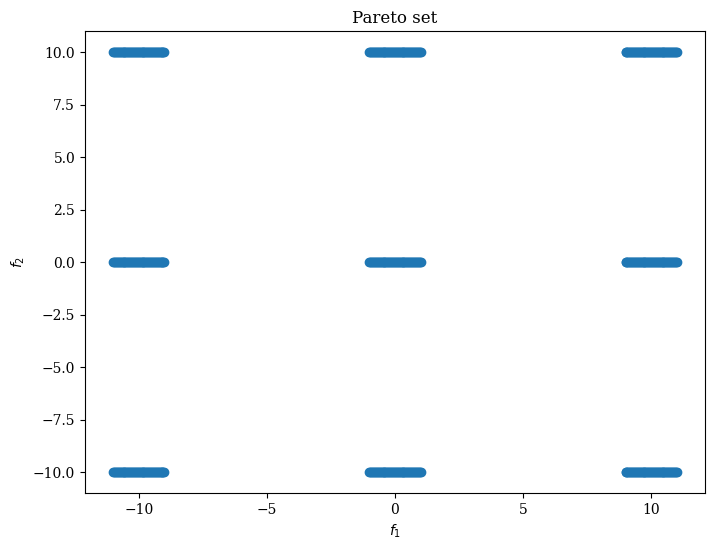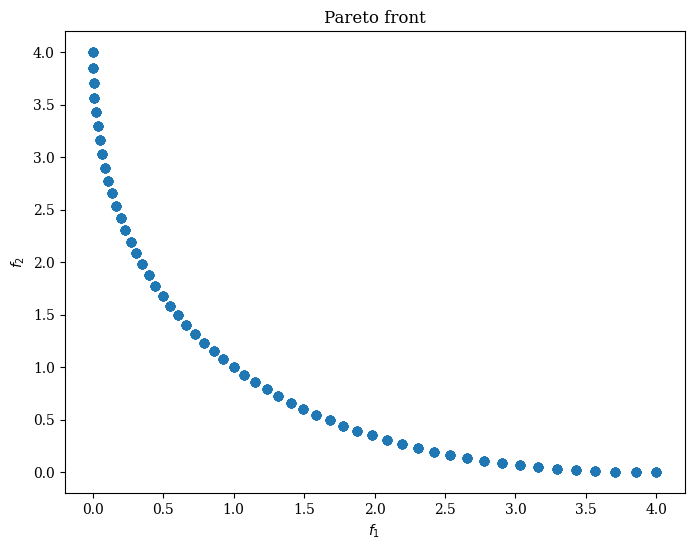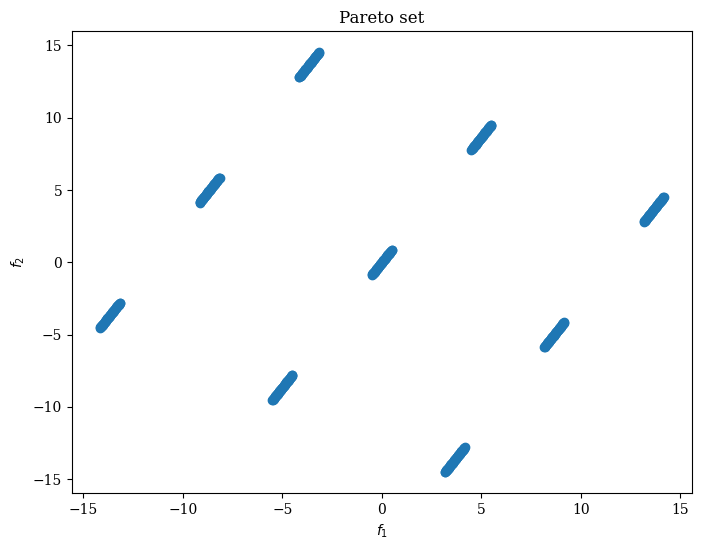Version: 0.5.0

# SYM-PART¶

The SYM-PART  problem suite is a multi-modal multi-objective optimization problem (MMOP). In MMOPs, a solution :math:y in the objective space may have several inverse images in the decision space. For this reason, an MMOP could have more than one Pareto subsets.

The SYM-PART has two variants: SYM-PART simple and SYM-PART rotated. Both of them have the same Pareto front. But their Pareto sets are different.

## 1. SYM-PART Simple¶

### Pareto subsets¶

:

from pymoo.problems.multi.sympart import SYMPART, SYMPARTRotated
from pymoo.visualization.scatter import Scatter

problem = SYMPART()
ps = problem.pareto_set()
Scatter(title="Pareto set", xlabel="$x_1$", ylabel="$x_2$").add(ps).show()

:

<pymoo.visualization.scatter.Scatter at 0x7f3fb9ab4550>### Pareto front¶

:

pf = problem.pareto_front()

:

<pymoo.visualization.scatter.Scatter at 0x7f3fb7956a60>## 2. SYM-PART Rotated¶

### Pareto subsets¶

The pareto subsets can be rotated.

:

from numpy import pi

# rotate pi/3 counter-clockwisely
problem = SYMPARTRotated(angle=pi/3)
ps = problem.pareto_set()
Scatter(title="Pareto set", xlabel="$x_1$", ylabel="$x_2$").add(ps).show()

:

<pymoo.visualization.scatter.Scatter at 0x7f3fb9ab4700>### Pareto front¶

:

pf = problem.pareto_front()

:

<pymoo.visualization.scatter.Scatter at 0x7f3fb71c9160>